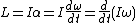# rotational motion

(redirected from Rotation around a fixed axis)
Also found in: Wikipedia.

## Rotational motion

The motion of a rigid body which takes place in such a way that all of its particles move in circles about an axis with a common angular velocity; also, the rotation of a particle about a fixed point in space. Rotational motion is illustrated by (1) the fixed speed of rotation of the Earth about its axis; (2) the varying speed of rotation of the flywheel of a sewing machine; (3) the rotation of a satellite about a planet; (4) the motion of an ion in a cyclotron; and (5) the motion of a pendulum. Circular motion is a rotational motion in which each particle of the rotating body moves in a circular path about an axis. Such motion is exhibited by the first and second examples. For information concerning the other examples See Harmonic motion, Particle accelerator, Pendulum

The speed of rotation, or angular velocity, remains constant in uniform circular motion. In this case, the angular displacement Θ experienced by the particle or rotating body in a time t is Θ = ωt, where ω is the constant angular velocity.

A special case of circular motion occurs when the rotating body moves with constant angular acceleration. If a body is moving in a circle with an angular acceleration of α radians/s2, and if at a certain instant it has an angular velocity ω0, then at a time t seconds later, the angular velocity may be expressed as ω = ω0 + αt, and the angular displacement as Θ = ω0t + ½αt2. See Acceleration, Velocity

A rotating body possesses kinetic energy of rotation which may be expressed as Trot = ½Iω2, where ω is the magnitude of the angular velocity of the rotating body and I is the moment of inertia, which is a measure of the opposition of the body to angular acceleration. The moment of inertia of a body depends on the mass of a body and the distribution of the mass relative to the axis of rotation. For example, the moment of inertia of a solid cylinder of mass M and radius R about its axis of symmetry is ½MR2.

The action of a torque L is to produce an angular acceleration α according to the equation below, where Iω, the productof moment of inertia and angular velocity, is called the angular momentum of the rotating body. This equation points out that the angular momentum Iω of a rotating body, and hence its angular velocity ω, remains constant unless the rotating body is acted upon by a torque. Both L and Iω may be represented by vectors.

It is readily shown that the work done by the torque L acting through an angle Θ on a rotating body originally at rest is exactly equal to the kinetic energy of rotation. See Angular momentum, Moment of inertia, Rigid-body dynamics, Torque, Work

McGraw-Hill Concise Encyclopedia of Physics. © 2002 by The McGraw-Hill Companies, Inc.

## rotational motion

[rō′tā·shən·əl ′mō·shən]
(computer science)
(fluid mechanics)
McGraw-Hill Dictionary of Scientific & Technical Terms, 6E, Copyright © 2003 by The McGraw-Hill Companies, Inc.
Site: Follow: Share:
Open / Close